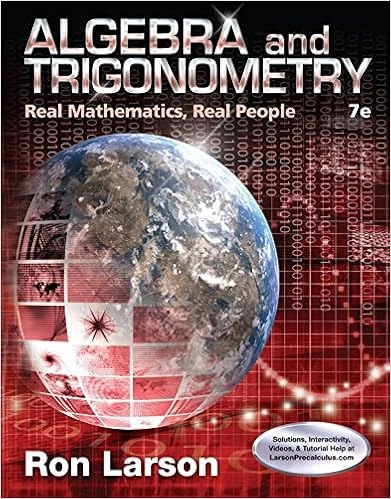# Use the following regression output to answer the

• Homework Help
• 10
• 100% (2) 2 out of 2 people found this document helpful

This preview shows page 2 - 5 out of 10 pages.

##### We have textbook solutions for you!
The document you are viewing contains questions related to this textbook.The document you are viewing contains questions related to this textbook.
Chapter 2 / Exercise 29
Algebra and Trigonometry: Real Mathematics, Real People
LarsonExpert Verified
Use the following regression output to answer the next 3 questions ___________ 2. TRUE OR FALSE. Two independent variables are statistically significant at = 0.05. : ___________ 3. TRUE OR FALSE. If another variable X4 is added to the regression, it is possible for R-Sq to decrease. A TRUE X2 and X3 are statistically significant at 5%.
___________ 4. What is the adjusted R-squared for this problem? D Adj-R-sq A) 0.0000 D) 0.4874 B) 0.0372 E) 0.5041 C) 0.3016 F) None of the above
##### We have textbook solutions for you!
The document you are viewing contains questions related to this textbook.The document you are viewing contains questions related to this textbook.
Chapter 2 / Exercise 29
Algebra and Trigonometry: Real Mathematics, Real People
LarsonExpert Verified
__________ 5. Suppose that P(A) = 0.2, P(B) = 0.2, and A is independent of B. What is P(A U B)? __________ 6. Which of the following statistics are always unitless? A) Slope D) Covariance D
B) Standard Error of the Regression E) None of the above. All have units C) Coefficient of Determination ___________ 7. A research and development engineer is preparing a report for the board of directors on the
expected life of a new mobile device they have produced. At a 95% confidence level, the engineer constructed the following interval for the average life of this new mobile device: 3.2 + 1 years. If the engineer would like to reduce the margin of error, which of the following would guarantee a smaller margin of error? Option (B) will decrease the critical value, making the margin of error smaller.
___________ 8. Which of the following statements is/are true about the t-distribution with k degrees of freedom? I. The t-distribution is symmetric II. The test for the significance of regression parameters as well as test for normality uses a t test. III. The t-distribution with k degrees of freedom has a variance greater than or equal to the t- distribution with k+1 degrees of freedom.
___________ 9. A data set consisting of 500 observations has a mean of 100, a median of 150, and a variance of 20. If the 500th observation which is equal to 100 was removed from the data set, what would happen to the variance of the data set? A) Removing the last observation would decrease the variance B) Removing the last observation would increase the variance C) Removing the last observation would keep the variance the same D) You need more information to determine whether the variance will change.
Use the following output to answer the next 2 questions : __________ 10. TRUE OR FALSE. X and Y must both be negatively skewed. __________ 11.
•••